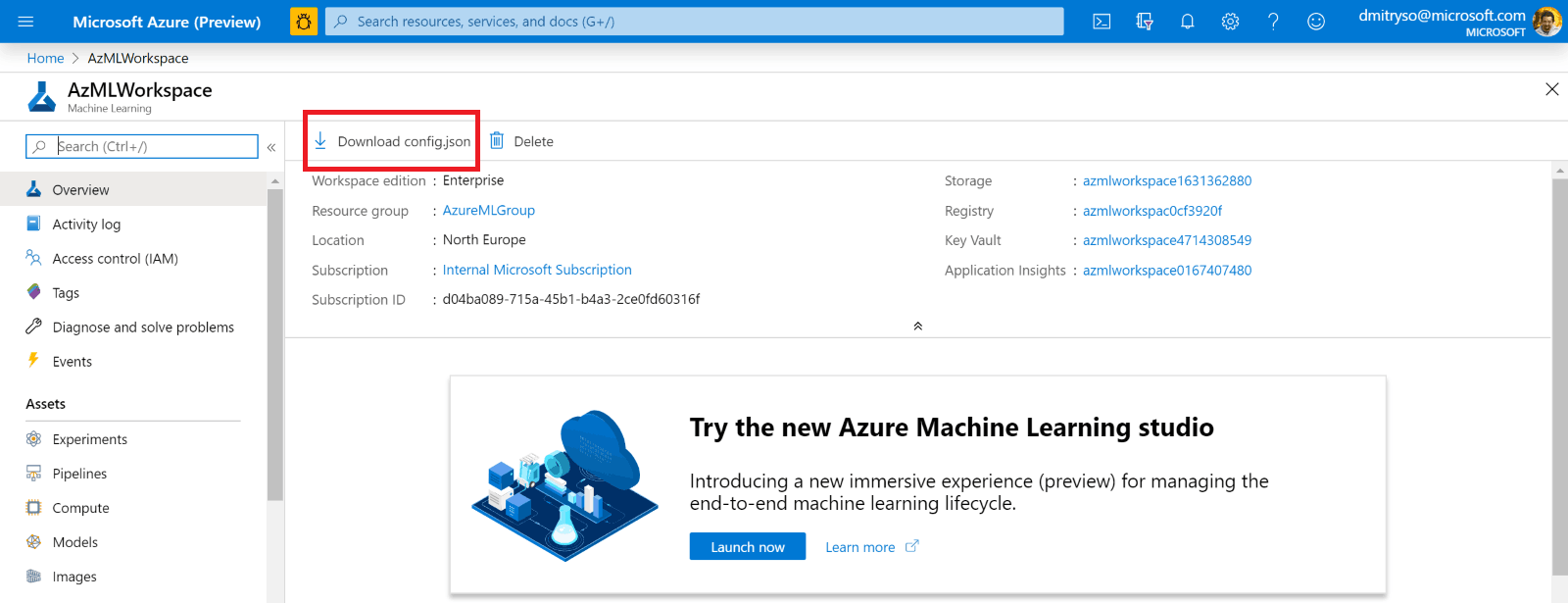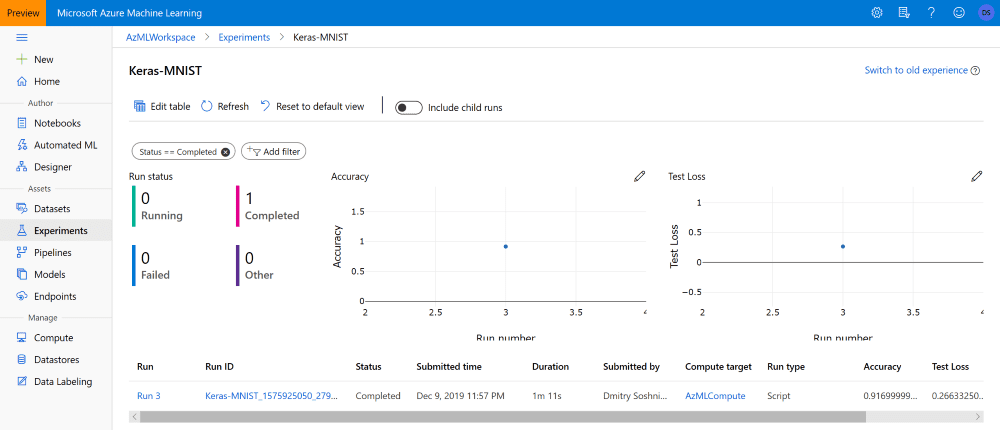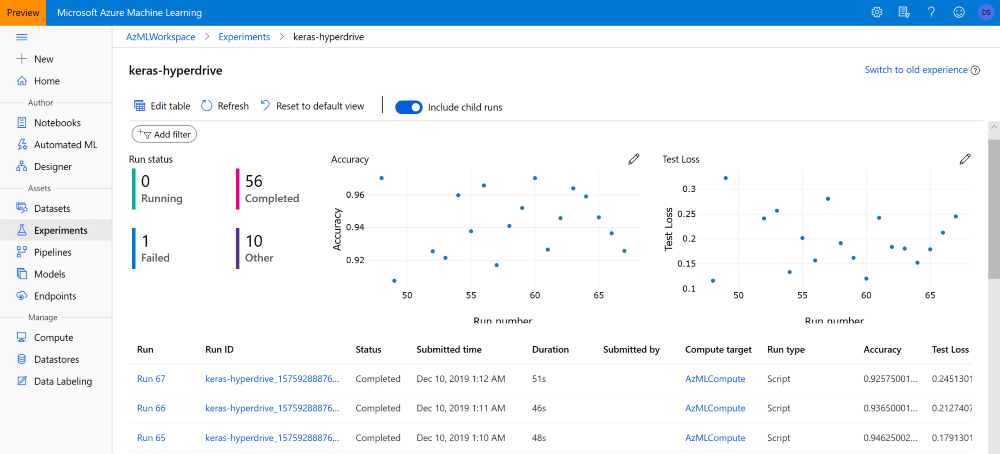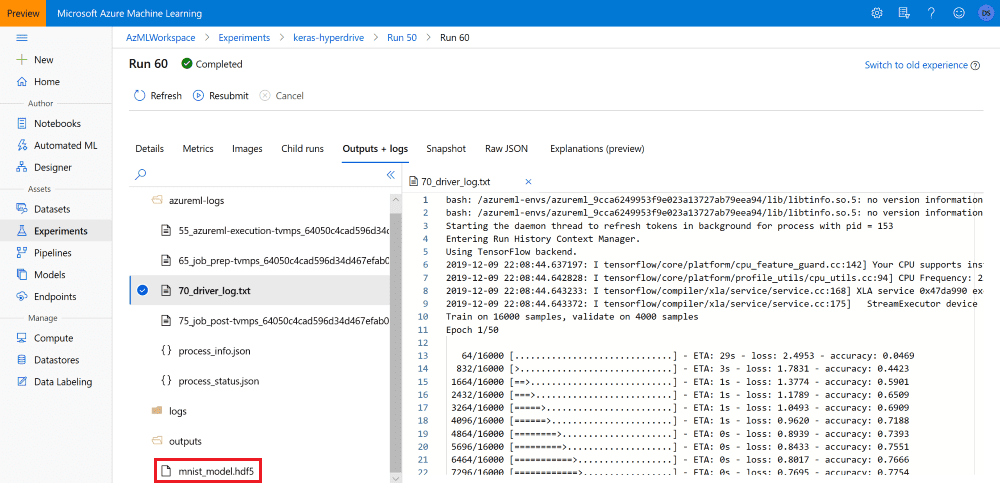# Using Azure Machine Learning for Hyperparameter Optimization

Most machine learning models are quite complex, containing a number of so-called hyperparameters, such as layers in a neural network, number of neurons in the hidden layers, or dropout rate. To build the best model, we need to chose the combination of those hyperparameters that works best. This process is typically quite tedious and resource-consuming, but Azure Machine Learning can make it much simpler.

In my previous post about Azure Machine Learning I have described how to start using Azure ML from Visual Studio Code. We will continue to explore the example described there, training simple model to do digit classification on MNIST dataset.

## Automation with Azure ML Python SDK

Hyperparameter optimization means that we need to perform large number of experiments with different parameters. We know that Azure ML allows us to accumulate all experiment results (including achieved metrics) in one place, Azure ML Workspace. So basically all we need to do is to submit a lot of experiments with different hyperparameters.

Instead of doing it manually from VS Code, we can do it programmatically through Azure ML Python SDK. All operations, including creating the cluster, configuring the experiment, and getting the results can be done with a few lines of Python code. This code can look a bit complex at first, but once you write (or understand) it once — you will see how convenient it is to use.

## Running the Code

The code that I refer to in this post is available in Azure ML Starter repository. Most of the code that I will describe here is contained inside `submit.ipynb` notebook. You can execute it in a few ways:

• If you have local Python environment installed, you can simply start local instance of Jupyter by running `jupyter notebook` in the directory with `submit.ipynb`. In this case, you need to install Azure ML SDK by running `pip install azureml-sdk`
• By uploading it to Notebook section in your Azure ML Portal and running it from there. You will also probably need to create a VM for executing notebooks from Azure ML Workspace, but that can be done from the same web interface quite seamlessly.

If you prefer working with plain Python files, the same code is available in submit.py as well.

## Connecting to Workspace and Cluster

The first thing you need to do when using Azure ML Python SDK is to connect to Azure ML Workspace. To do so, you need to provide all required parameters such as subscription id, workspace and resource group name more info on docs:

``````ws = Workspace(subscription_id,resource_group,workspace_name)
``````

The easiest way to connect is to store all required data inside `config.json` file, and then instantiate the workspace reference like this:

``````ws = Workspace.from_config()
``````

You can download `config.json` file from your Azure Portal, by navigating to the Azure ML Workspace page:Once we have obtained the workspace reference, we can get the reference to the compute cluster that we want to use:

``````cluster_name = "AzMLCompute"
cluster = ComputeTarget(workspace=ws, name=cluster_name)
``````

This code assumes that you have already created the cluster manually (as described in the previous post). You can also create the cluster with required parameters programmatically, and the corresponding code is provided in submit.ipynb.

In our MNIST training example, we downloaded MNIST dataset from the OpenML repository on the Internet inside the training script. If we want to repeat the experiment many times, it would make sense to store the data somewhere close to the compute — inside the Azure ML Workspace.

First of all, let’s create MNIST dataset as a file on disk in `dataset` folder. To do that, run `[create_dataset.py](https://github.com/CloudAdvocacy/AzureMLStarter/blob/master/create_dataset.py)` file, and observe how `dataset` folder is created, and all data files are stored there.

Each Azure ML Workspace has a default datastore associated with it. To upload our dataset to the default datastore, we need just a couple of lines of code:

``````ds = ws.get_default_datastore()
``````

## Submitting Experiments Automatically

In this example, we will train the two-layer neural network model in Keras, using train_keras.py training script. This script can take a number of command-line parameters, which allow us to set different values for hyperparameters of our model during training:

• `--data_folder`, that specifies path to the dataset
• `--batch_size` to use (default is 128)
• `--hidden`, size of the hidden layer (default is 100)
• `--dropout` to use after the hidden layer

To submit the experiment with given parameters, we first need to create `Estimator` object to represent our script:

``````script_params = {
'--data_folder': ws.get_default_datastore(),
'--hidden': 100
}

est = Estimator(source_directory='.',
script_params=script_params,
compute_target=cluster,
entry_script='train_keras.py',
pip_packages=['keras','tensorflow'])
``````

In this case, specified just one hyperparameter explicitly, but of course we can pass any parameters to the script to train the model with different hyperparameters. Also, note that estimator defines pip (or conda) packages that need to be installed in order to run our script.

Now, to actually execute the experiment, we need to run:

``````exp = Experiment(workspace=ws, name='Keras-Train')
run = exp.submit(est)
``````

You can then monitor the experiment right inside the notebook by printing our `run` variable (it is recommended to have `azureml.widgets` extension installed in Jupyter, if you are running it locally), or by going to the Azure ML Portal:## Hyperparameter Optimization using HyperDrive

Optimization of hyperparameters involves some sort of parametric sweep search, which means that we need to run many experiments with different combinations of hyperparameters and compare the results. This can be done manually using the approach we have just discussed, or it can be automated using the technology called Hyperdrive.

In Hyperdrive, we need to define search space for hyperparameters, and the sampling algorithm, which controls the way hyperparameters are selected from that search space:

``````param_sampling = RandomParameterSampling({
'--hidden': choice([50,100,200,300]),
'--batch_size': choice([64,128]),
'--epochs': choice([5,10,50]),
'--dropout': choice([0.5,0.8,1])})
``````

In our case, the search space is defined by a set of alternatives (`choice`), while it is also possible to use continuos intervals with different probability distributions (`uniform`, `normal`, etc. – more details here). In addition to Random Sampling, it is also possible to use Grid Sampling (for all possible combinations of parameters) and Bayesian Sampling.

In addition, we can also specify Early Termination Policy. It makes sense if our script reports metrics periodically during the execution – in this case we can detect that accuracy being achieved by a particular combination of hyperparameters is lower than median accuracy, and terminate the training early:

``````early_termination_policy = MedianStoppingPolicy()
hd_config = HyperDriveConfig(estimator=est,
hyperparameter_sampling=param_sampling,
policy=early_termination_policy,
primary_metric_name='Accuracy',
primary_metric_goal=PrimaryMetricGoal.MAXIMIZE,
max_total_runs=16,
max_concurrent_runs=4)
``````

Having defined all the parameters for a hyperdrive experiment, we can submit it:

``````experiment = Experiment(workspace=ws, name='keras-hyperdrive')
hyperdrive_run = experiment.submit(hd_config)
``````

In the Azure ML Portal, hyperparameter optimization is represented by one experiment. To view all results on one graph, select include child runs checkbox:## Chosing the Best Model

We can compare the results and select the best model manually in the portal. In our training script train_keras.py, after training the model, we stored the result into `outputs` folder:

``````hist = model.fit(...)
os.makedirs('outputs',exist_ok=True)
model.save('outputs/mnist_model.hdf5')
``````

Having done this, we can now locate the best experiment in the Azure ML Portal and get the corresponding `.hdf5` file to be used in inference:Alternatively, we can use Azure ML Model Management to register the model, which would allow us to keep better track of it, and use it during Azure ML Deployment. We can programmatically find the best model and register it using the following code:

``````best_run = hyperdrive_run.get_best_run_by_primary_metric()
best_run_metrics = best_run.get_metrics()
print('Best accuracy: {}'.format(best_run_metrics['Accuracy']))
best_run.register_model(model_name='mnist_keras',
model_path='outputs/mnist_model.hdf5')
``````

## Conclusion

We have learnt how to submit Azure ML Experiments programmatically via Python SDK, and how to perform Hyperparameter optimization using Hyperdrive. While it takes a while to get used to this process, you will soon realize that Azure ML simplifies the process of model tuning, comparing to doing it “by hand” on a Data Science Virtual Machine.

AZURE
ai azure machine learning azureml